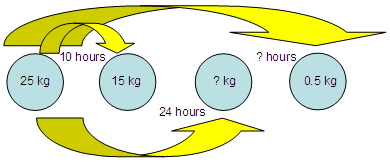# Exponential decay – Half life formula

### Decay calculator in Matlab

 A quantity is said to be subject to exponential decay if it decreases at a rate proportional to its value. Half-life is the period of time it takes for a substance undergoing decay to decrease by half. Half-lives are very often used to describe quantities having exponential decay, where the half-life is constant over the whole life of the decay, and is a natural unit of scale for the exponential decay formula.

The formulas below are used in calculations involving the exponential decay of, for example, radioactive materialsLet’s code a Matlab function to calculate the final amount of a substance, given the elapsed time, half-life and initial amount. This is just a code of the ‘final amount’ formula above

function end_amt = exp_decay(beg_amt, hl, time)
% beg_amt = beginning amount
% hl = half life
% time = elapsed time

n = time / hl;
end_amt = beg_amt/2^n;

This is a possible code to calculate the half-life of a substance, if we’re given the initial and final amounts, and the elapsed time between those masses:

function hl = half_life(beg_amt, fin_amt, time)
% beg_amt = beginning amount
% fin_amt = final amount
% time = elapsed time

hl = log10(2) * time / log10(beg_amt/fin_amt);

And this code calculates the time needed for a substance undergoing exponential decay to go from an initial mass to a final mass. We need to know the half-life, naturally.

function t = elapsed_time(beg_amt, fin_amt, hl)
% beg_amt = beginning amount
% fin_amt = final amount
% hl = half life

t = hl * log10(beg_amt / fin_amt) / log10(2);

Now, let’s test our decay calculators to discover ending amounts: if we start with 100 grams of radium, and we know its half-life is 1620 years, how much would be left after 3240 years (two half-lives)?

We can type this from the command window or from any other script. It’s important to follow the order of the parameters:

end_amt = exp_decay(100, 1620, 3240)

Matlab shows that the ending amount would be 25, as expected.

If 10 grams are present now, how much will be present in 50 years?

end_amt = exp_decay(10, 1690, 50)

The result on screen is end_amt = 9.7970

Example:

Let’s suppose that salt decomposes in water into chloride and sodium ions according to the law of exponential decay. If the initial amount of salt is 25 kg and after 10 hours, 15 kg of salt is left, how much salt is left after 1 day? How long does it take until 0.5 kg of salt is left?

We need to understand what we’re being asked. We can make a drawing to understand better...We could create an m-file named ‘test_exp_decay’, for example, and we’d type this:

clear, clc, format compact

% Initial amount of salt is 25 kg
% after 10 hours, 15 kg of salt is left

beg_amt = 25;
fin_amt = 15;
time = 10;
hl = half_life(beg_amt, fin_amt, time)

% How much salt is left after 1 day?
time = 24;
end_amt = exp_decay(beg_amt, hl, time)

% How long does it take until 1/2 kg of salt is left?
fin_amt = 0.5;
t = elapsed_time(beg_amt, fin_amt, hl)

The code represents the given problem and its solution. First, we solve for the half-life; second, we solve for the ending amount; finally, we solve for the elapsed time considered in the formulas.